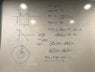# Electric field between two capacitor plates (proof)

Harry17
Homework Statement:
I’m trying to prove that the electric field strength between 2 capacitor plates is uniform. To do this, I first considered a capacitor consisting of 2 circular disks, with charges +Q and -Q respectively, and determined a charge per unit area, and used that to find the charge on concentric rings going outwards from the centre of the plate. Taking the integral of the force from each ring as a function of x (the distance from the centre of the plate) and r (the distance from one of the plates) gives an integral which I am not yet able to integrate but subbing values in and using a calculator to give me a value shows that it does depend on r, when it should not.

Not sure where I’ve gone wrong, a photo of my working is attached. Any advice or solutions would be greatly appreciated.

Many thanks
Relevant Equations:
F=Qq(Kd^2)
See attached image

#### Attachments

•image.jpg
57.7 KB · Views: 156

Homework Helper
The glare makes your expressions hard to read. Am I right if I suspect you are adding two vector magnitudes when you should be subtracting them ?

Harry17
The forces act in the same direction so I assumed that I add them? The two terms come from the forces from the left hand side plate and the right hand side plate

•